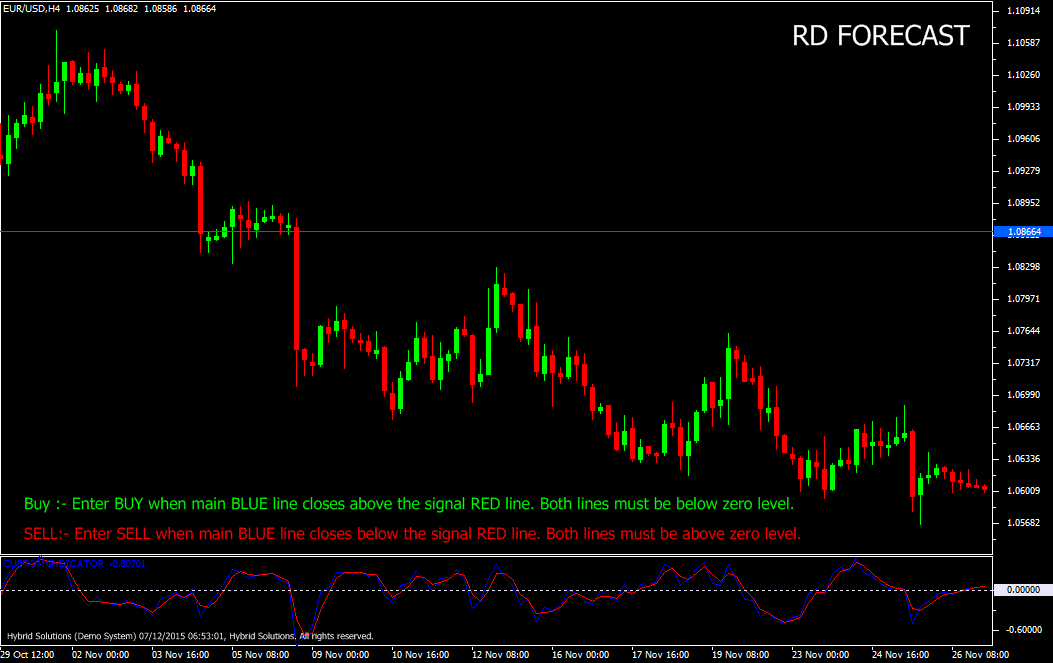# RD Forecast

Free

Developed By: NeeduVerdeSolution

In stock

RD-Forecast is a powerful VertexFX client side VTL oscillator that provides zero-lag identification of market cycles and turning points. Because of its low lag, it is often called the forecast oscillator.

It is based on the concept of weighted smoothing and regression. At first step, we calculate the various regression and smoothing constants based for the regression period T3 and the smoothing constant B. In second step, we calculate the Linear Weighted Moving average of the closing prices over the regression period. The nearest candle has the highest weight multiplier, and the farthest candle has the lowest weight multiplier.Now, we calculate the main BLUE indicator value by smoothing it six times using the smoothing co-efficients e1, e2, e3, e4, e5 and e6 calculated in step one.

SKU: 859 Categories: ,RD-Forecast is a powerful VertexFX client side VTL oscillator that provides zero-lag identification of market cycles and turning points. Because of its low lag, it is often called the forecast oscillator.

It is based on the concept of weighted smoothing and regression. At first step, we calculate the various regression and smoothing constants based for the regression period T3 and the smoothing constant B. In second step, we calculate the Linear Weighted Moving average of the closing prices over the regression period. The nearest candle has the highest weight multiplier, and the farthest candle has the lowest weight multiplier.Now, we calculate the main BLUE indicator value by smoothing it six times using the smoothing co-efficients e1, e2, e3, e4, e5 and e6 calculated in step one.

The signal RED is calculated by smoothing the main BLUE indicator, using the smoothing constant of B. The concept behind this indicator is that, by giving a higher weightage to the current price over the previous and farther prices, we are able to identify the trend with higher accuracy (lower error factor) using linear regression. Using the smoothing co-efficient e1, e2, e3, e4, e5 and e6 , the lag is eliminated and we get a better quality of the signal.

Buy: Place Buy position when main Blue line closes above the signal RED line. Both lines must be below zero level. Do not enter trade if candle has opened with a gap-up.

Sell: Enter SELL when main BLUE line closes below the signal RED line. Both lines must be above zero level. Do not enter trade if candle has opened with a gap-down.